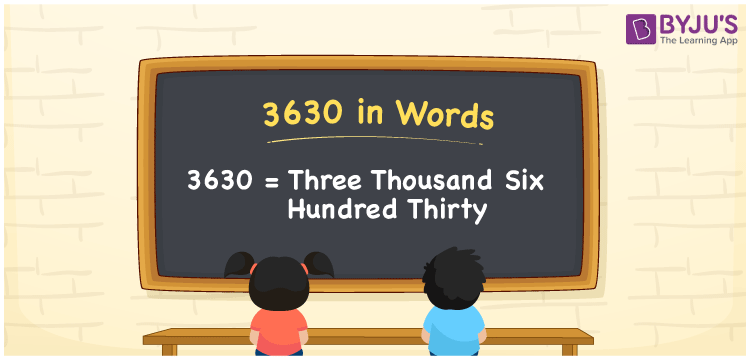# 3630 in words

3630 in words is written as Three Thousand Six Hundred and Thirty. 3630 represents the count or value. The article on Counting Numbers can give you an idea about count or counting. The number 3630 is used in expressions that relate to money, distance, length, year and others. Let us consider an example for 3630. ”I have travelled Three Thousand Six Hundred and Thirty kilometres in the last week.”

 3630 in words Three Thousand Six Hundred and Thirty Three Thousand Six Hundred and Thirty in Numbers 3630

## 3630 in English Words## How to Write 3630 in Words?

We can convert 3630 to words using a place value chart. The number 3630 has 4 digits, so let’s make a chart that shows the place value up to 4 digits.

 Thousands Hundreds Tens Ones 3 6 3 0

Thus, we can write the expanded form as:

3 × Thousand + 6 × Hundred + 3 × Ten + 0 × One

= 3 × 1000 + 6 × 100 + 3 × 10 + 0 × 1

= 3630

= Three Thousand Six Hundred and Thirty.

3630 is the natural number that is succeeded by 3629 and preceded by 3631.

3630 in words – Three Thousand Six Hundred and Thirty.

Is 3630 an odd number? – No.

Is 3630 an even number? – Yes.

Is 3630 a perfect square number? – No.

Is 3630 a perfect cube number? – No.

Is 3630 a prime number? – No.

Is 3630 a composite number? – Yes.

## Solved Example

1. Write the number 3630 in expanded form

Solution: 3 × 1000 + 6 × 100 + 3 × 10 + 0 × 1

We can write 3630 = 3000 + 600 + 30 + 0

= 3 × 1000 + 6 × 100 + 3 × 10 + 0 × 1

## Frequently Asked Questions on 3630 in words

Q1

### How to write 3630 in words?

3630 in words is written as Three Thousand Six Hundred and Thirty.
Q2

### Is 3630 a perfect square number?

No. 3630 is not a perfect square number.
Q3

### Is 3630 a prime number?

No. 3630 is not a prime number.